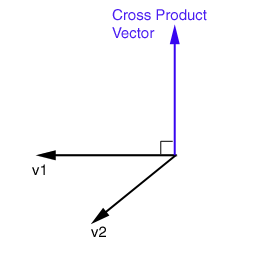Prev: Vector Length Next: Dot Product

The cross product takes two vectors and returns a perpendicular vector to the plane defined by the two vectors. Assuming that v1 and v2 are in the XZ plane, then the direction of the cross product vector will point upwards if the rotation from v1 to v2 is counter clockwise, and downwards if the rotation is clockwise. Its usage includes, amongst other things, lighting computations, see the terrain tutorial, or to find the angle between vectors.The equations bellow show the necessary steps to compute the cross product v, of two vectors v1 and v2. The operation is commonly represented by “x”.

```	v = v1 x v2

v = [vx,vy,vz] where,

vx = v1y * v2z - v1z * v2y

vy = v1z * v2x - v1x * v2z

vz = v1x * v2y - v1y * v2x```

Assuming that v1 and v2 are in the XZ plane, then the direction of the cross product vector will point upwards if the rotation from v1 to v2 is counter clockwise, and downwards if the rotation is clockwise.

Note that the cross product operation is not commutative, it is in fact anti-commutative. Bellow is a list of some properties of the cross product:

```	v1 x v2 = - (v2 x v1)
k * (v1 x v2) = v1 x (k*v2) = (k*v1) x v2
v1 x (v2 + v3) = (v1 x v2) + (v1 x v3)```

The length of the cross product vector is proportional to v1 and v2 length, as well as the sine of the angle between v1 and v2.

`       |v| = |v1| |v2| * sin(a)`

where a is the angle between the two vectors. As shown in the above formula, the cross product can also be used to compute the sine of the angle between two vectors (see the inner product for the cosine). The cross product can be defined as a C macro:

```#define crossProduct(a,b,c) \

(a) = (b) * (c) - (c) * (b); \
(a) = (b) * (c) - (c) * (b); \
(a) = (b) * (c) - (c) * (b);```

 Prev: Vector Length Next: Dot Product

This site uses Akismet to reduce spam. Learn how your comment data is processed.